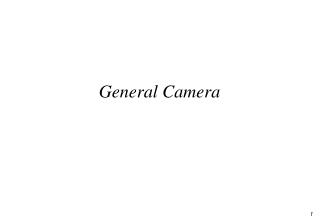DownloadDownload PresentationGeneral Camera

# General Camera

Download Presentation## General Camera

- - - - - - - - - - - - - - - - - - - - - - - - - - - E N D - - - - - - - - - - - - - - - - - - - - - - - - - - -
##### Presentation Transcript

1. General Camera

2. Overview • Simple camera is limiting and it is necessary to model a camera that can be moved • We will define parameters for a camera in terms of where it “is”, the direction it points and the direction it considers to be “up” on the image

3. Simple Camera (Cross Section) Y d ymax Z -Z COP ymin

4. General Camera • View Reference Point (VRP) • where the camera is • View Plane Normal (VPN) • where the camera points • View Up Vector (VUV) • which way is up to the camera • X (or U-axis) forms LH system

5. UVN Coordinates • View Reference Point (VRP) • origin of VC system (VC=View Coordinates) • View Plane Normal (VPN) • Z (or N-axis) of VC system • View Up Vector (VUV) • determines Y (or V-axis) of VCS • X (or U-axis) forms Left Hand system

6. Y V U V U V V R P N X Z World Coords and Viewing Coords (EQ1) We want to find a general transform of the above form (EQ1) that will map WC to VC

7. View from the Camera N and VPN into the page V xmax, ymax VUV Z Y X U xmin, ymin

8. Finding the basis vectors • Step 1 - find n • Step 2 - find u • Step 3 - find v

9. Finding the Mapping (1) • u,v,n must rotate under R to i,j,k of viewing space • Both basis are normalised so this is a pure rotation matrix • recall in this case RT = R-1

10. Finding the Mapping (2) • In uvn system VRP (q) is (0 0 0 1) • And we know from EQ1 so

11. Complete Mapping • Complete matrix

12. For you to check • If • Then

13. Using this for Ray-Casting • Use a similar camera configuration (COP is usually, but not always on -n) • To trace object must either • transform spheres into VC • transform rays into WC

14. Ray-casting • Transforming rays into WC • Transform end-point once • Find direction vectors through COP as before • Transform vector by • Intersect spheres in WC

15. Ray-casting • Transforming spheres into VC • Centre of sphere is a point so can be transformed as usual (WC to VC) • Radius of sphere is unchanged by rotation and translation (and spheres are spheroids if there is a non-symmetric scale)

16. Tradeoff • If more rays than spheres do the former • transform spheres into VC • For more complex scenes e.g. with polygons • transform rays into WC

17. Alternative Forms of the Camera • Simple “Look At” • Give a VRP and a target (TP) • VPN = TP-VRP • VUV = (0 1 0) (i.e. “up” in WC) • Field of View • Give horizontal and vertical FOV or one or the other and an aspect ratio • Calculate viewport and proceed as before

18. Animated Cameras • Animate VRP (observer-cam) • Animate VPN (look around) • Animate TP (track-cam) • Animate COP • along VPN - zoom • orthogonal to VPN - distort

19. Recap • We created a more general camera which we can use to create views of our scenes from arbitrary positions • Formulation of mapping from WC to VC (and back)